#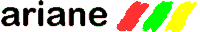Aero Formulas

Here are some aero formulas which might come in handy on occasion. Note that (in order to be able to use the same GIFs for both english and german versions of this page) commas are used for a decimal point, also the terminology is german - such as A (Auftrieb) for Lift, W (Widerstand) for Drag, Flügel=Wing, Rumpf=Fuse, etc.

Lift in stationary glide:Lift coefficient:Drag coefficient:Moment coefficient:Reynolds number:simplified: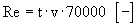Drag coefficient of a flat plate, laminar flow:Drag coefficient of a flat plate, turbulent flow:Combined drag coefficient, general:Airplane drag coefficient:Fuse surface area:where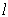= length and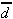= mean diameter.

Fuse drag coefficient:(Hoerner)

Aspect ratio: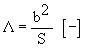Glide ratio:Glide angle:Mean chord of a trapeziod wing:Turn radius:G load:Energy loss due to drag: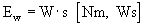Altitude loss due to drag:Power loss due to drag:Climb rate in powered flight:Flight speed:Sink speed: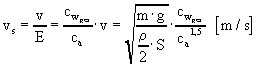Horizontal stab factor: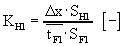where= stab lever arm (distance of the t/4 lines)

Lift coefficient of horizontal stab: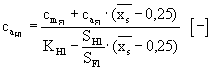where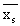= location of C.G.

Induced angle of attack:Induced drag coefficient:k = correction factor for wing geometry (0 for an elliptic planform with no washout)

List of Symbols: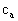Lift coefficient [-]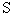Area [m˛]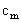Moment coefficient [-]Flight distance [m]Drag coefficient [-]Chord [m]Lift [N]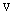Speed [m/s]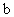Wingspan [m]Drag [N]Glide ratio [-]Air density 1,225 kg/mł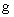Gravitational constant 9,81 m/s˛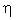Dynamical viscosity 0,0000174 Pa·s (Air, 15°C)Momentum [Nm]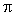Pi 3,1415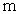Mass [kg]Aspect ratio [-]Reynolds number [-]

Home | Up | DEUTSCH    (Hannes Delago - Apr 8, 1997)